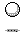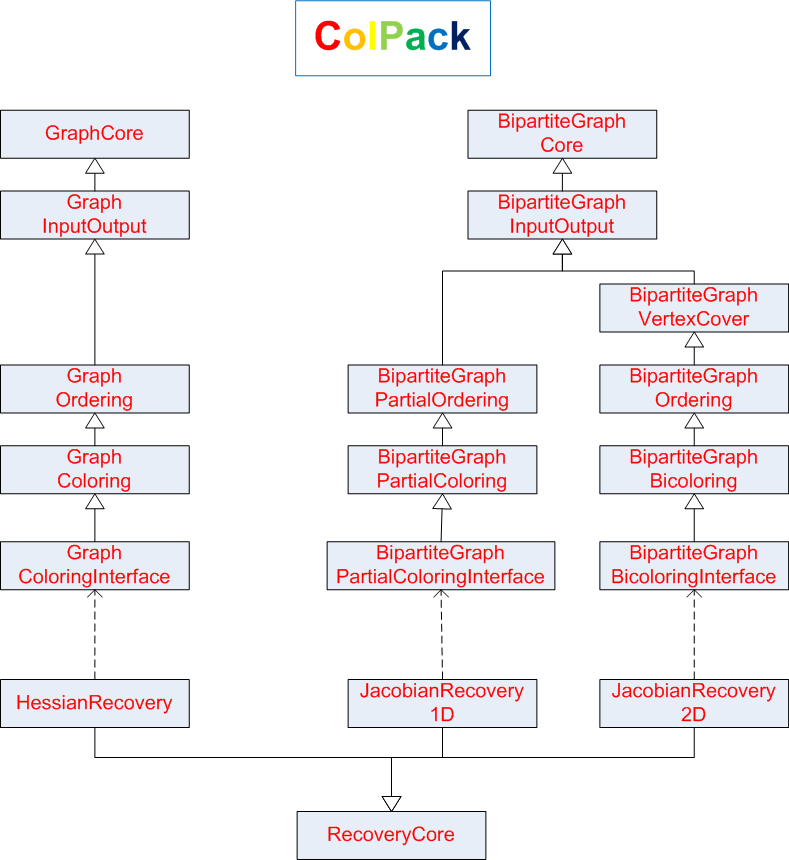Sparse Derivative Computation NSF and DOE Funded Project Home | People | Papers | Presentations |Software | Automatic Differentiation

Content
This page contains a brief discussion of the background for, the functionalities available in, and the organization of our serial coloring software package ColPack. For a detailed discussion of the algorithms on which the package relies, consult the Papers page.

Sparse derivative computation and coloring

Four-step procedure

The computation of an m-by-n sparse derivative matrix A using automatic differentiation (AD) can be made efficient by using the following four-step procedure.

1. Determine the sparsity structure of A.
2. Using a specialized vertex coloring on an appropriate graph representation of the matrix A, obtain an n-by-p seed matrix S that defines a partitioning of the columns of A into p groups with p as small as possible.
3. Compute the compressed matrix B = AS using AD.
4. Recover the numerical values of the entries of A from B.

The set of criteria used to define the seed matrix S in the second step, the partitioning problem on the matrix A, depends on three mutually orthogonal factors:

• Whether the derivative matrix being computed is a Jacobian (nonsymmetric) or a Hessian (symmetric),
• Whether the numerical values of the entries of the original matrix A are obtained from the compressed representation B directly (without any further arithmetic) or indirectly (for example, by solving for unknowns via successive substitution), and
• Whether the matrix partitioning is unidirectional (involving only columns or only rows) or bidirectional (involving both columns and rows). The four-step procedure above is described assuming a column-wise unidirectional partitioning. In a row-wise unidirectional partitioning (which is a better approach for Jacobian matrices with a few dense rows), the compressed matrix would correspond to the seed-matrix-Jacobian product STA. Similarly, in a bidirectional partitioning (which might be the best approach for Jacobian matrices with both a few dense rows and a few dense columns), the Jacobian entries are recovered from two compressed matrices S1TA and AS2.

Coloring models

Table 1 below provides a summary of the most accurate coloring models for the various computational scenarios. In each case, the structure of a Jacobian matrix A is represented by the bipartite graph Gb(A) = (V1, V2, E), where the vertex sets V1 and V2 represent the rows and columns of A, respectively, and each nonzero matrix entry Aij is represented by the edge (ri , cj) in E. Analogously, the structure of a Hessian matrix A is represented by the adjacency graph G(A) = (V, E), where the vertex set V represents the columns (or, by symmetry, the rows) of A and each off-diagonal nonzero matrix entry Aij  (and its symmetric counterpart Aji) is represented by the single edge (ci, cj) in E.

 1d partition 2d partition Jacobian Partial distance-2 coloring Star bicoloring Direct Hessian Star coloring NA Direct Jacobian NA Acyclic bicoloring Substitution Hessian Acyclic coloring NA Substitution

Table 1: Overview of coloring models in derivative computation. NA stands for not applicable.

In a graph G = (V, E), two distinct vertices are distance-k neighbors if a shortest path connecting them consists of at most k edges. A distance-k coloring of the graph is an assignment of positive integers (called colors) to the vertices such that every two distance-k neighboring vertices get different colors. A star coloring is a distance-1 coloring where, in addition, every path on four vertices uses at least three colors. An acyclic coloring is a distance-1 coloring in which every cycle uses at least three colors. The names star and acyclic coloring are due to the structures of two-colored induced subgraphs: a collection of stars in the case of star coloring and a collection of trees in the case of acyclic coloring.

In a bipartite graph Gb = (V1, V2, E), a partial distance-2 coloring on the vertex set Vi, i = 1,2, is an assignment of colors to the vertices in Vi such that any two vertices connected by a path of length exactly two edges receive different colors. Star and acyclic bicoloring in a bipartite graph are defined in a manner analogous to star and acyclic coloring in a general graph, but with the additional stipulation that the set of colors assigned to row vertices (V1) is disjoint from the set of colors used for column vertices (V2).

ColPack : functionalities

ColPack is a package comprising of implementations of algorithms for the specialized vertex coloring problems discussed in the previous section as well as algorithms for a variety of related supporting tasks in derivative computation.

Coloring capabilities

Table 2 below gives a quick summary of all the coloring problems (on general and bipartite graphs) supported by ColPack.

 General Graph G = (V, E) Bipartite Graph Gb = (V1, V2, E): One-sided Coloring Bipartite Graph Gb = (V1, V2, E): Bicoloring ·        Distance-1 coloring     O(|V|∙d1)  = O(|E|) ·          Partial distance-2 coloring on V2        O(|V2|· d(V2) · Δ(V1)) = O (|E|·Δ(V1)) ·        Star bicoloring† O((|V1|+ |V2|)∙d2)) ·        Distance-2 coloring     O(|V|∙d2) ·          Partial distance-2 coloring on V1         O(|V1|· d(V1) · Δ(V2))  = O(|E|·Δ(V2)) ·        Star coloring†                     O(|V|∙d2) ·        Acyclic coloring                O(|V|∙d2∙α) ·        Restricted star coloring        O(|V|∙d2) ·        Triangular coloring†      O(|V|∙d2)

Table 2: List of coloring problems for which implementations of algorithms are available in ColPack. Problems with the superscript have more than one algorithm implemented in ColPack; the complexity listed in each case is that of the fastest algorithm.

All of the coloring problems listed in Table 2 are NP-hard. Their corresponding algorithms in ColPack are greedy heuristics in the sense that the algorithms progressively extend a partial coloring by processing one vertex at a time, in some order, in each step assigning a vertex the smallest allowable color. Listed beneath each coloring problem in Table 2 is the complexity of the corresponding algorithm in ColPack. In the cases where ColPack has multiple algorithms for a problem (these are designated by the superscript ), the complexity expression corresponds to that of the fastest algorithm. In the complexity expressions,

• dk denotes the average degree-k, the number of distinct paths of length at most k edges leaving a vertex. Thus d1(v) corresponds to the usual degree of  the vertex v, the number of edges incident on v, and d2(v) corresponds to the sum of the degree-1 values of the vertices adjacent to v.
• α denotes the inverse of Ackermann’s function.
• d(Vi) and Δ(Vi) denote the average and maximum, respectively, vertex degree-1 in the set Vi, i=1,2, of the bipartite graph Gb = (V1, V2 , E).

Ordering techniques

The order in which vertices are processed in a greedy coloring algorithm determines the number of colors used by the algorithm. ColPack has implementations of various effective ordering techniques for each of the supported coloring problems. These are summarized in Table 3 below.

 General Graph Bipartite Graph: One-sided Coloring Bipartite Graph: Bicoloring ·        Natural ·        Column Natural ·        Natural ·        Largest First ·        Column Largest First ·        Largest First ·        Smallest Last ·        Column Smallest Last ·        Smallest Last ·        Incidence Degree ·        Column Incidence Degree ·        Incidence Degree ·        Dynamic Largest First ·        Row Natural ·        Dynamic Largest First ·        Distance-2 Largest First ·        Row Largest First ·        Selective Largest First ·        Distance-2 Smallest Last ·        Row Smallest Last ·        Selective Smallest Last ·        Distance-2 Incidence Degree ·        Row Incidence Degree ·        Selective Incidence Degree ·        Distance-2 Dynamic Largest First

Recovery routines

Besides coloring and ordering capabilities, ColPack also has routines for recovering the numerical values of the entries of a derivative matrix from a compressed representation. In particular the following reconstruction routines are currently available:

• Recovery routines for direct (via star coloring ) and substitution-based (via acyclic coloring) Hessian computation
• Recovery routines for unidirectional, direct Jacobian computation (via column-wise or row-wise distance-2 coloring)
• Recovery routines for bidirectional, direct Jacobian computation via star bicoloring

Graph construction routines

Finally, as a supporting functionality, ColPack has routines for constructing bipartite graphs (for Jacobians) and adjacency graphs (for Hessians) from files specifying matrix sparsity structures in various formats, including Matrix Market, Harwell-Boeing and MeTis.

ColPack : organization

ColPack is written in an object-oriented fashion in C++ heavily using the Standard Template Library (STL).  It is designed to be simple, modular, extensible and efficient. Figure 1 below gives an overview of the structure of the major classes of ColPack.Figure 1: Overview of the structure of the major classes in ColPack. A solid arrow indicates an inheritance-relationship, and a broken arrow indicates a uses-relationship.

ColPack functions that a user needs to call directly are made available via the appropriate Interface classes.

Sample Codes

The following sample codes illustrate how ColPack functions are called in the context of sparse derivative computation via the Four-step Procedure. In each sample code, the de-compressed sparse derivative matrix is returned in the Coordinate Format (zero-based indexing). Recovery routines that return the de-compressed matrix in Direct Sparse Solver and ADOL-C Formats are also available in ColPack.

Column-wise Jacobian Computation (via partial distance-2 coloring)

Row-wise Jacobian Computation (via partial distance-2 coloring)

Direct Hessian Computation (via star coloring)

Indirect Hessian Computation (via acyclic coloring)

Bidirectional, direct Jacobian Computation (via star bicoloring)

Here is the source code of ColPack.  It is being distributed under the GNU Lesser General Public License.

And here are a few test graphs for experiments.

Graph Collection in MeTis format